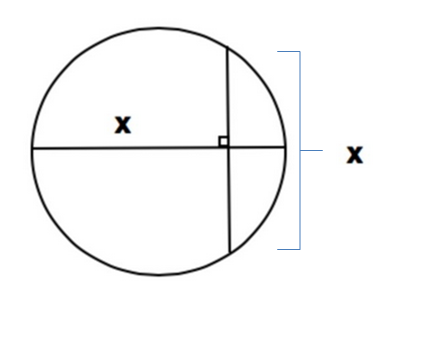# Chord-Diameter Intersection

Geometry Level 3If the circle above has a diameter of 1, and $x$ can be written in the form $\dfrac{a}{b}$, where $a$ and $b$ are coprime integers. Find the value of $a+b$.

×